An attempt to find a generalized approach for shuffling a very large list that does not fit in memory. I explore the creation of generators that sample items without repeats using random number generators.

# Problem Overview

For one of my projects, I need to generate a random permutation (without repetition) of the integers from 0 to n. In my particular situation, I am generating permutations for large n and I am only using a small portion of the values at the beginning of sampled permutation (e.g., generating a permutation of a shorter length k, where k is small but n is large). My initial approach was to build an array of values from 0 to n and then shuffling it using the Fisher-Yates algorithm. However, for large values of n, I am unable to hold all of the elements in memory (in Java, I get a heap overflow), so I started looking for more memory efficient approaches that let me generate the elements of a random permutation as needed. In essence, I am looking for an algorithm to do a lazy list shuffle.

I did some googling and found this approach, which consists of constructing a finite field given a prime modulus m; e.g., $$aX$$ mod $$m$$ for $$a = 1,2,3, \cdots, n$$. We know that the field contains the numbers $$1 \cdots m-1$$ when m is prime (I think it was Gauss that showed this in his book Disquisitiones Arithmeticae). This ensures that we generate all of the values in the desired range at some point. However, sometimes it generates additional values (when m is larger than the size of the range). In these cases, we can simply ignore values outside the range and keep generating values until they are in the desired range. This approach, which comes from the work on format-preserving encryption</a>, is actually pretty efficient if m is not that much larger than the size of the range because only a small portion of values are invalid. Thus, this approach works by selecting the smallest prime $$m$$ larger than the size of the range we want to generate, selecting a random generator $$a$$, and selecting an initial seed $$X_0$$. Then we can generate successive values using the relation $$X_n = X_{n-1} + a$$ mod $$m$$. While this approach can be used to lazily generate the elements of a random permutation with constant memory, some of my early testing showed that the permutations generated are not actually that random.

To overcome this limitation, I started looking for alternative approaches that generate more “random” looking permutations. It turns out the first approach I found is a special case of a linear congruential generator, a well-known pseudorandom number generator, that only has an additive component (no multiplicative component). Researching this type of number generator more, I found that a Lehmer, or multiplicative congruential, generator is another special case of a linear congruential generator that can be used to generate more random looking permutations. This type of generator lets me have precise control over the number of elements in the finite field, which I can use to ensure we generate full permutations. Additionally, I found another pseudorandom number generator, a linear feedback shift register, that can be used to generate more random looking sequences, while still having precise control over the number of elements in the finite field. These two approaches, which are both based on Finite Field theory, require the selection of a modulus and a generator that determines the size of the finite field and the order in which these elements are generated. To ensure that the finite fields in both approaches have maximal order (i.e., that all of the values in the range appear in the generated permutation) we must ensure that the generators in both approaches are primitive roots of the finite fields. For the multiplicative congruential generator, this consists of selecting the smallest prime $$m$$ larger than the size of the range and a value $$a$$ that is a primitive root modulo $$m$$. For the linear feedback shift register, this consists of selecting a modulo two polynomial that is a primitive root for the number of bits being used in the linear feedback shift register.

To test all three approaches, I implemented them in python (I refer to the functions as lazyshuffledrange1, lazyshuffledrange2, and lazyshuffledrange3) and compare them to each other and to the naive Fisher-Yates approach (which I call shuffledrange). Here are my python implementations of each function.

# Approaches

## Evaluation

Before conducting any evaluation, a simple analysis of the algorithms shows that the three new functions use constant memory because we don’t retain any of the elements of the permutations and simply use the last value in the permutation sequence to generate the next. This is substantially better than the shuffledrange function that generates the entire range in memory and shuffles it with the Fisher-Yates algorithm. Next, I wanted to get a sense of how random each approach is, so I plotted the output of each function for generating a permutation of the values from $$0 \cdots 100$$. Here are the four plots: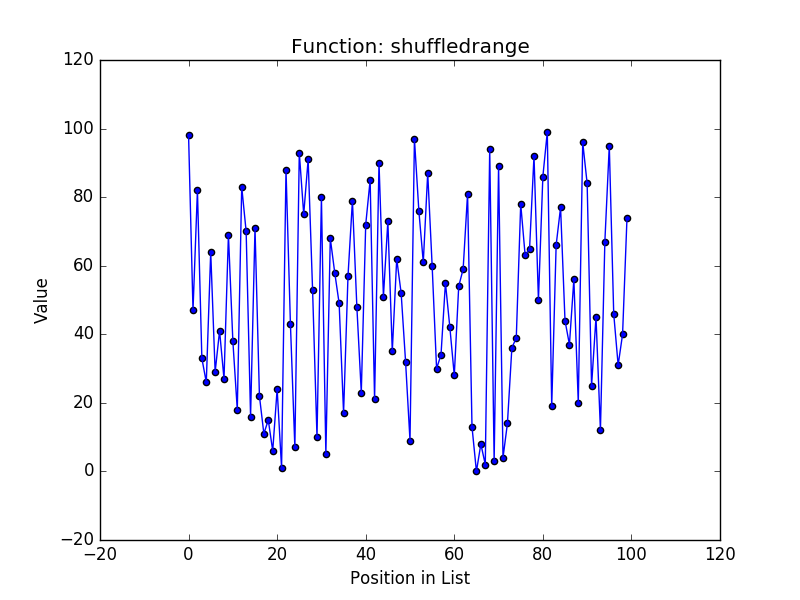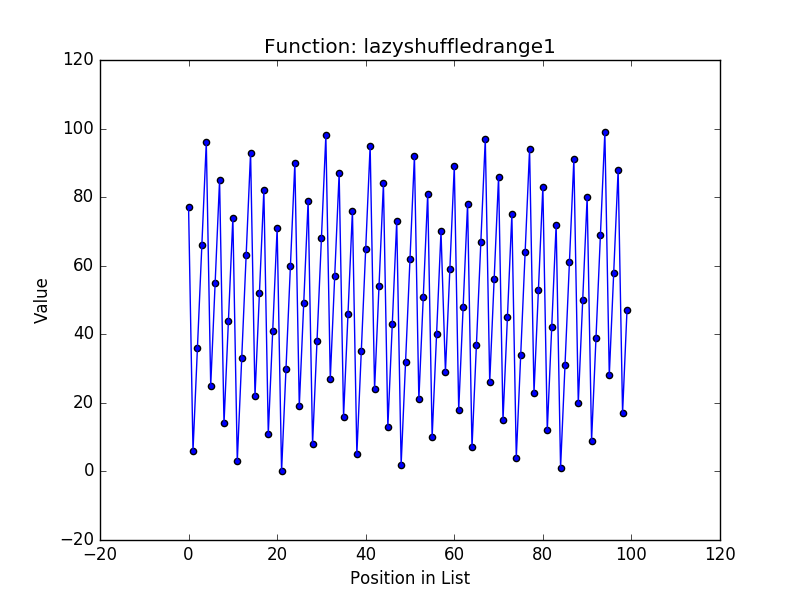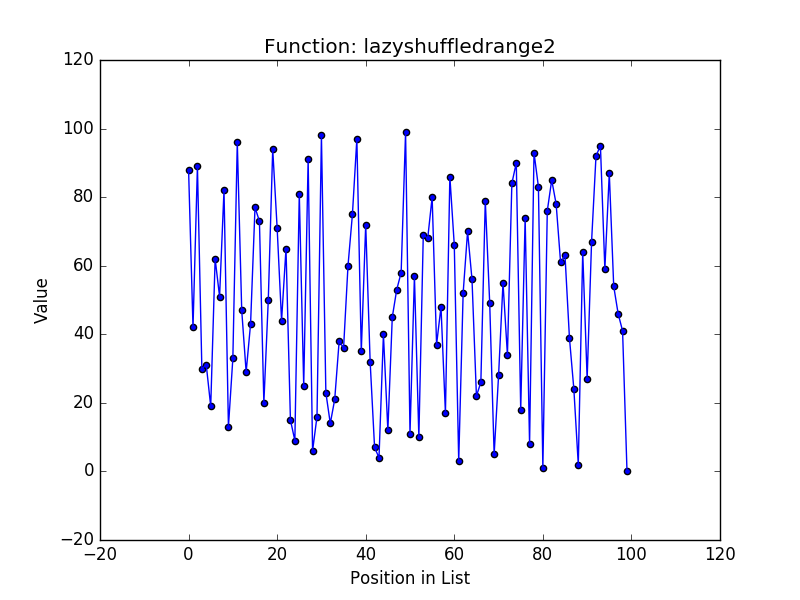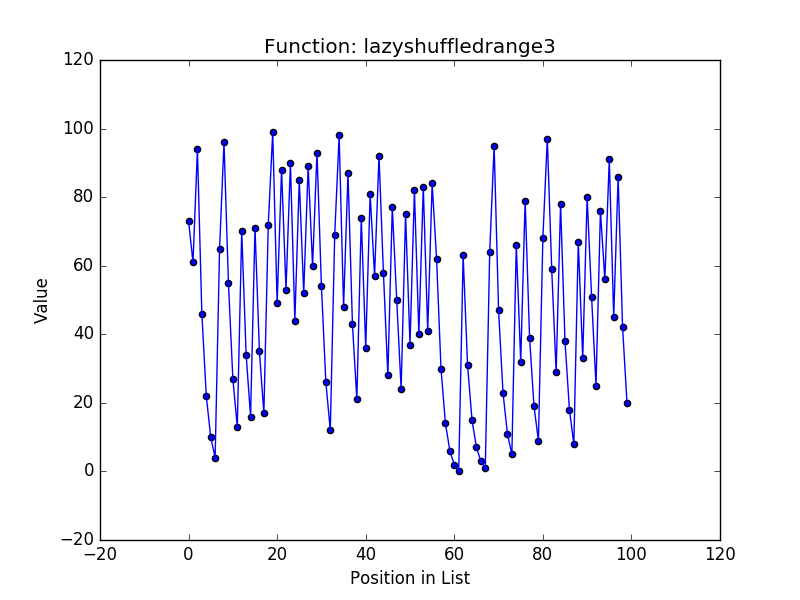These plots show that the most random outputs seem to be the shuffledrange (Fisher-Yates is known to generate each possible permutation with equal likelihood) and lazyshuffledrange2 (the multiplicative congruential generator). The lazyshuffledrange3 function (the linear feedback shift register) also seems to be random, but it seems to have some values grouped together. Finally, the lazyshuffledrange1 (the additive congruential generator) is clearly not random (this is the result I was mentioning earlier that led me to try the two additional approaches).

Next, I tested how fast each of these approaches is. To do this, I used python’s “timeit” function to test how quickly each function could generate a permutation of the values $$0 \cdots 1000$$. As an additional check of the randomness, I measured the spearman correlation of the generated values with their position in the list. For both the timing and correlation tests, I ran each approach 3 times and picked the fastest of the three runs, as well as the lowest correlation, to get a sense of best case for each approach. Here are the results:

Shuffle Function Time (sec) Spearman r pval
shuffledrange 10.779 -0.085 0.007
lazyshuffledrange1 3.833 -0.032 0.305
lazyshuffledrange2 5.527 -0.043 0.170
lazyshuffledrange3 10.834 -0.015 0.636

Next, to get a sense of how these approaches scale, I timed each function for ranges of increasing size. Here is a timing data plotted for each algorithm for ranges of size 10, 100, 1000, and 10000: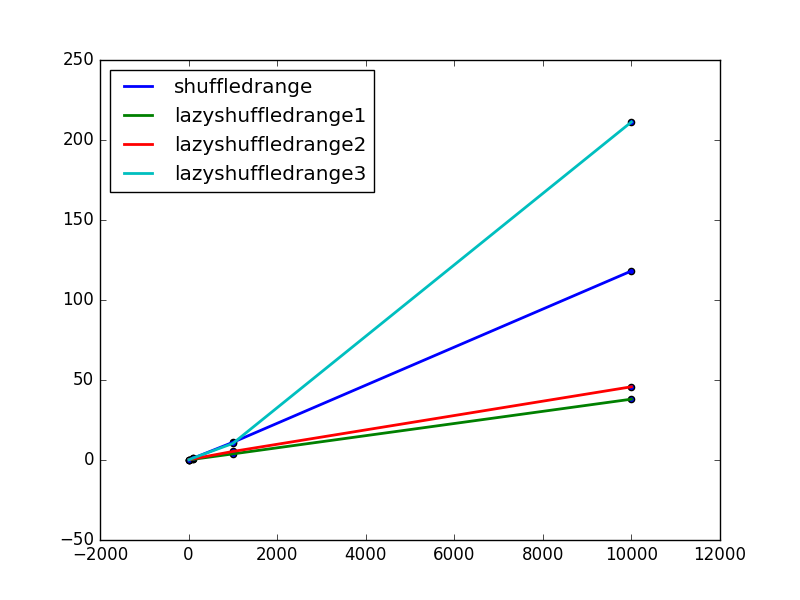These results show that the lazyshuffledrange1 (the additive congruential generator) and lazyshuffledrange2 (the multiplicative congruential generator) functions are the fastest and seem to scale well with increase in the size of the range. In contrast, lazyshuffledrange3 (the linear feedback shift register) is slower than the shuffledrange (the Fisher-Yates) function. This is likely because my implementation of lazyshuffledrange3 uses precomputed primitive roots for mods that are powers of 2. So the number of values outside the range that are still in the finite field tend to be large, so the function takes more iterations to generate valid values.

As a final test, I wanted to see if repeated calls to each algorithm were uniformly sampling from the space of possible permutations. To evaluate this, I chose a small range ($$0 \cdots 6$$) and called each function 1000 times given this range. Here I plotted the histograms over the resulting permutations: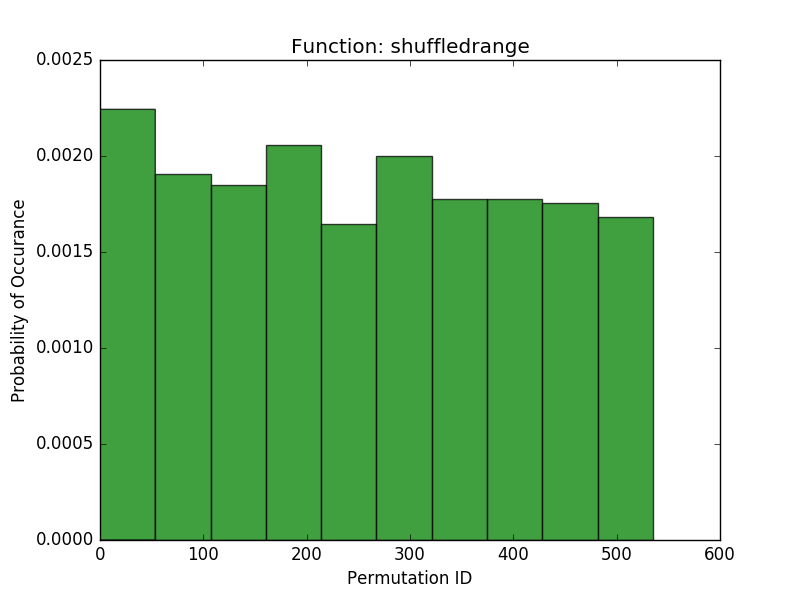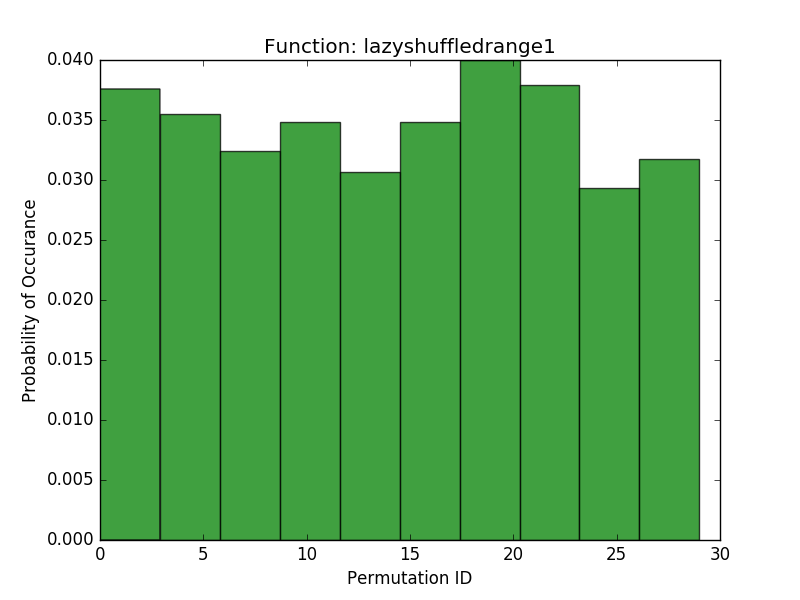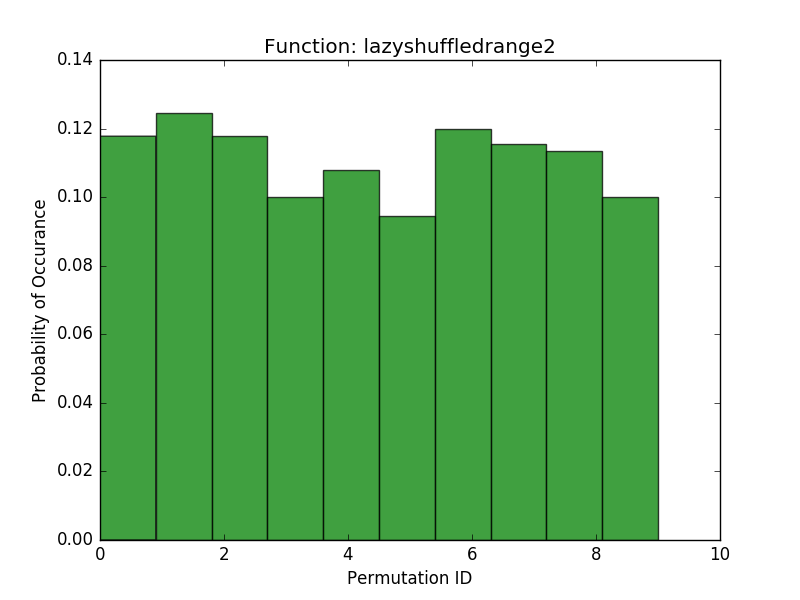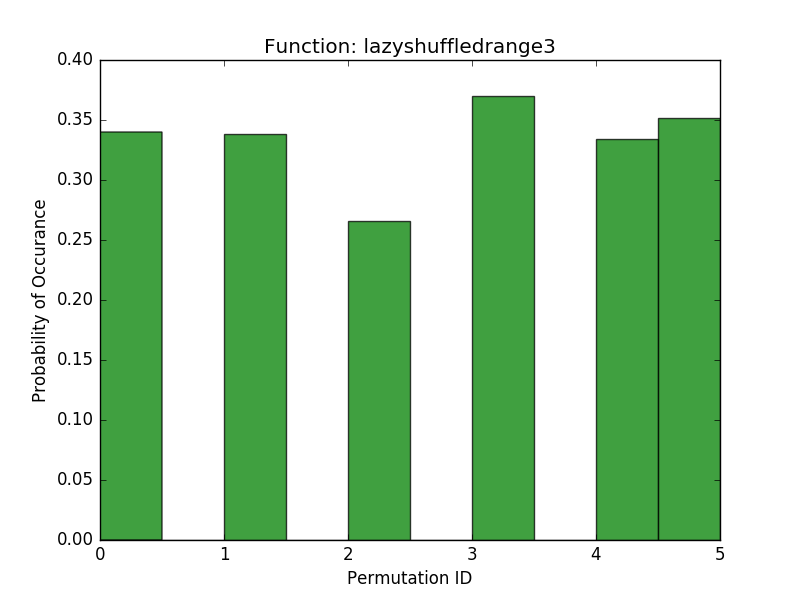These results show that the shuffledrange (Fisher-Yates) seems to approach a uniform probability of each permutation (although the pydocs make note that for large n, not all permutations may be possible). Our more memory efficient approaches seem to have a pretty uniform probability of each permutation that they generate, but they are all limited in the number of permutations they can generate. The lazyshuffledrange1 (the additive congruential generator) seems to generate the most permutations, and lazyshuffledrange3 (the linear feedback shift register) generates the smallest number of permutations. Lazyshuffledrange2 (the multiplicative congruential generator) is somewhere in the middle. If one is interested in generating the full space of possible permutations, then this is a troublesome result. On the other hand, the results do make some sense. The number of permutations generated corresponds roughly to the number of possible states for the generators. For example, lazyshuffledrange1 accepts a generator $$a$$ between 1 and the modulus and a seed between 1 and the size of the range. If our range is length 6, then the modulus is 7 (the smallest prime larger than 6) and $$a \in [2, 6]$$ and $$seed \in [0,5]$$, so there are 30 possible initial conditions, which agrees with the number of permutations we see in the histogram. By contrast, the lazyshuffledrange2 function also uses the modulus 7 (the smallest prime larger than the size of the range), but $$a \in \{3, 5\}$$ (there are only 2 primitive roots mod 7, see this table) and $$seed \in [1,5]$$ (a multiplicative congruential generator cannot start off at 0), so there are only 10 possible initial conditions, which agrees with the histogram results. Finally, the linear feedback shift register has fixed primitive roots (because I used a prebuilt table), so its only parameter is $$seed \in [0,5]$$, or 6 initial conditions, which also agrees with the histogram results. In order to support more possible permutations we could increase the prime modulus of these generators until the number of possible permutations is large enough. However, this will take more computing power (because more of the values in the finite field are outside the range and more iterations will need to be performed to get values within the range).

As a basic test of this idea, I increased the modulus of the lazyshuffledrange2 function to be the smallest prime larger than 2 times the size of the range, which resulted in the following histogram: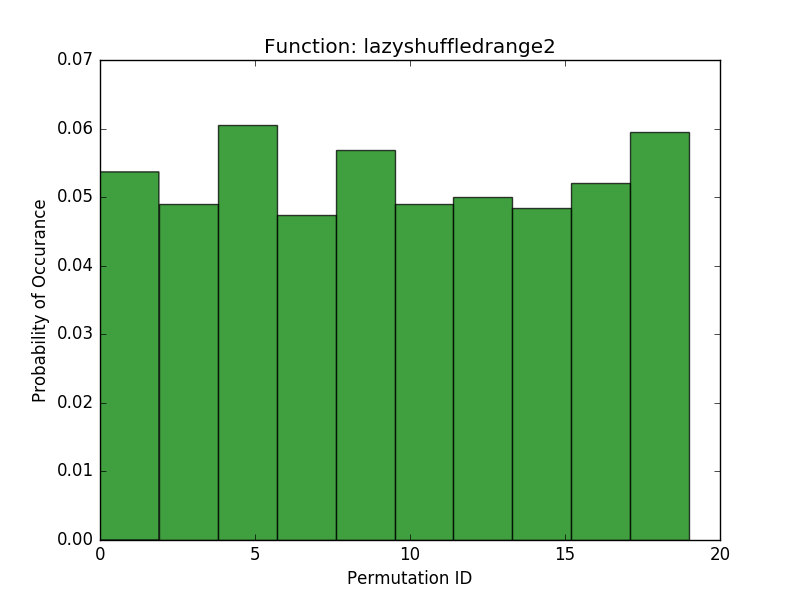As we can see, this doubling of the number of possible states in the generator yields approximately a doubling in the number of permutations it can generate.

# Summary

I implemented four algorithms, one is the naive Fisher-Yates shuffle (shuffledrange) and the other three are more memory efficient approaches to lazily generating the values of a random shuffle (i.e., permutation without repetition) of a list. These approaches are the additive congruential generator (lazyshuffledrange1), multiplicative congruential generator (lazyshuffledrange2), and the linear feedback shift register (lazyshuffledrange3). All three approaches are based on finite field theory. I found that the fastest approach that also (by my very naive eye-ball test and basic correlation analysis) seems to generate suitably pseudorandom shufflings was the multiplicative congruential generator. It also seems to have uniform probability over the possible permutations it can generate. However, the number of possible permutations it can produce is limited by the number of possible states that can be represented in the multiplicative congruential generator, which is determined by the modulus of the finite field. If more possible permutations are needed, then the modulus can be increased. This results in more possible permutations that can be generated at an additional computation cost. In situations where only a few permutations are needed, then it is probably a sufficient memory efficient approach to lazily generating the elements of a shuffled list.

If any readers have thoughts on how I can increase the possible permutations that can be generated by any of my proposed approaches, I would love to hear about it!